# Riemann integral

A generalization of the concept of a Cauchy integral to a certain class of discontinuous functions; introduced by B. Riemann (1853). Consider a function $f$ which is given on an interval $[a,b]$. Let $a=x_0<x_1<\dots<x_n=b$ is a partition (subdivision) of the interval $[a,b]$ and $\Delta x_i = x_i-x_{i-1}$, where $i=1,\dots,n$. The sum \begin{equation}\label{eq:1} \sigma = f(\xi_1)\Delta x_1+\dots+f(\xi x_i)\Delta x_i +\dots +f(x_n)\Delta x_n, \end{equation} where $x_{i-1}\leq\xi_i\leq x_i$, is called the Riemann sum corresponding to the given partition of $[a,b]$ by the points $x_i$ and to the sample of points $\xi_i$. The numberis called the limit of the Riemann sums (1) asif for anya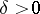can be found such that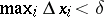implies the inequality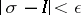. If the Riemann sums have a finite limitas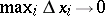, then the functionis called Riemann integrable over, where. The limit is known as the definite Riemann integral ofover, and is written as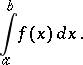(2)

When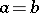then, by definition,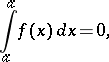and whenthe integral (2) is defined using the equation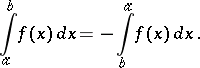A necessary and sufficient condition for the Riemann integrability ofoveris the boundedness ofon this interval and the zero value of the Lebesgue measure of the set of all points of discontinuity ofcontained in.

## Properties of the Riemann integral.

1) Every Riemann-integrable functiononis also bounded on this interval (the converse is not true: The Dirichlet function is an example of a bounded and non-integrable function on).

2) The linearity property: For any constantsand, the integrability overof both functionsandimplies that the function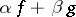is integrable over this interval, and the equation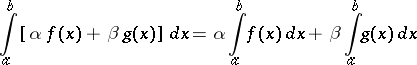holds.

3) The integrability overof both functionsandimplies that their product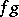is integrable over this interval.

4) Additivity: The integrability of a functionover both intervals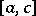and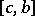implies thatis integrable over, and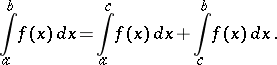5) If two functionsandare integrable overand iffor everyin this interval, then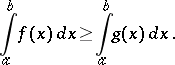6) The integrability of a functionoverimplies that the function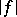is integrable over this interval, and the estimate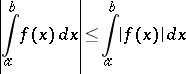holds.

7) The mean-value formula: If two real-valued functionsandare integrable over, if the functionis non-negative or non-positive everywhere on this interval, and ifandare the least upper and greatest lower bounds ofon, then a numbercan be found,, such that the formula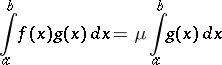(3)

holds. If, in addition,is continuous on, then this interval will contain a pointsuch that in formula (3),8) The second mean-value formula (Bonnet's formula): If a functionis real-valued and integrable overand if a functionis real-valued and monotone on this interval, then a pointcan be found insuch that the formula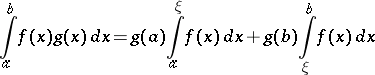holds.